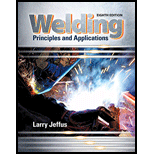Chapter 20, Problem 14R### Welding: Principles and Applicatio...

8th Edition
Larry Jeffus
ISBN: 9781305494695

#### Solutions

Chapter
Section### Welding: Principles and Applicatio...

8th Edition
Larry Jeffus
ISBN: 9781305494695
Textbook Problem
3 views

# What would the labor cost be if 20 hours were worked at an hourly rate of $25? To determine Total labor cost. Explanation Given information: Number of hours worked, H=20hours. Rate of the labor, R=$25 per hour.

Calculation:

Total labor cost is the multiplication of rate of the labor and the number of days or hours work has done,

C=numberof

### Still sussing out bartleby?

Check out a sample textbook solution.

See a sample solution

#### The Solution to Your Study Problems

Bartleby provides explanations to thousands of textbook problems written by our experts, many with advanced degrees!

Get Started

#### Define an electrolyte and its role in battery operation.

Automotive Technology: A Systems Approach (MindTap Course List)

#### Convert 10,000 ft-lbf of energy into BTU, Joules, and kilojoules.

Fundamentals of Chemical Engineering Thermodynamics (MindTap Course List)

#### What is the relationship between mass flow rate and volume flow rate?

Engineering Fundamentals: An Introduction to Engineering (MindTap Course List)